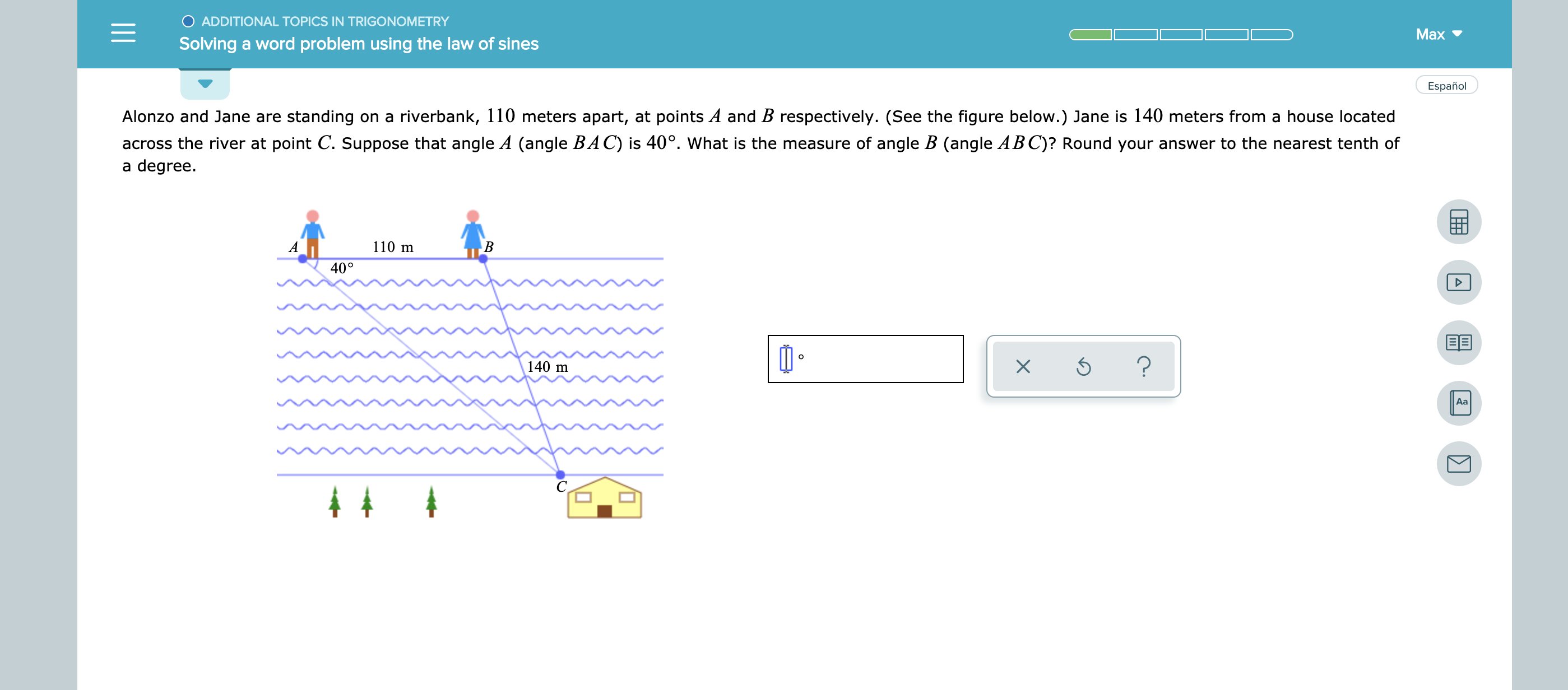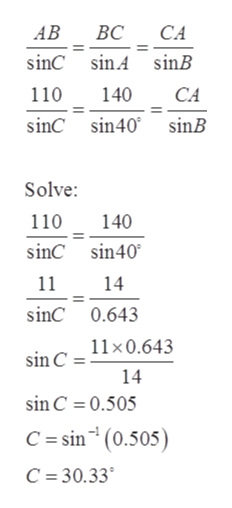# ADDITIONAL TOPICS IN TRIGONOMETRYMaxSolving a word problem using the law of sinesEspañolAlonzo and Jane are standing on a riverbank, 110 meters apart, at points A and B respectively. (See the figure below.) Jane is 140 meters from a house locatedacross the river at point C. Suppose that angle A (angle BAC) is 40°. What is the measure of angle B (angle ABC)? Round your answer to the nearest tenth ofa degree110 mAВ40°?140 mAaX-

Question
1 views

see attachedhelp_outlineImage TranscriptioncloseADDITIONAL TOPICS IN TRIGONOMETRY Max Solving a word problem using the law of sines Español Alonzo and Jane are standing on a riverbank, 110 meters apart, at points A and B respectively. (See the figure below.) Jane is 140 meters from a house located across the river at point C. Suppose that angle A (angle BAC) is 40°. What is the measure of angle B (angle ABC)? Round your answer to the nearest tenth of a degree 110 m A В 40° ? 140 m Aa X - fullscreen
check_circle

Step 1

Consider the given figure.

Step 2

Formula used: Sine rule,

Step 3

Now, applying the sine rul...help_outlineImage TranscriptioncloseCA АВ ВС sinC sin A sinB 110 140 CA sinC sin40 sinB Solve 110 140 sinC sin40 11 14 sinC 0.643 11x0.643 sin C 14 sin C 0.505 C = sin (0.505) C 30.33 fullscreen

### Want to see the full answer?

See Solution

#### Want to see this answer and more?

Solutions are written by subject experts who are available 24/7. Questions are typically answered within 1 hour.*

See Solution
*Response times may vary by subject and question.
Tagged in

### Trigonometry